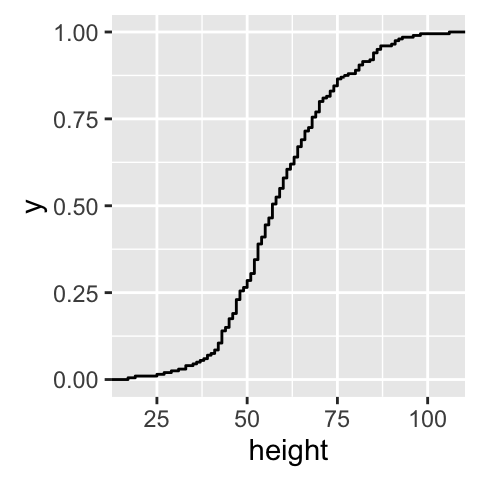# ggplot2 ECDF plot : Quick start guide for Empirical Cumulative Density Function - R software and data visualization

This R tutorial describes how to create an ECDF plot (or Empirical Cumulative Density Function) using R software and ggplot2 package. ECDF reports for any given number the percent of individuals that are below that threshold.

The function stat_ecdf() can be used.

# Create some data

``````set.seed(1234)
df <- data.frame(height = round(rnorm(200, mean=60, sd=15)))
``````##   height
## 1     42
## 2     64
## 3     76
## 4     25
## 5     66
## 6     68``````

# ECDF plots

``````library(ggplot2)
ggplot(df, aes(height)) + stat_ecdf(geom = "point")
ggplot(df, aes(height)) + stat_ecdf(geom = "step")``````For any value, say, height = 50, you can see that about 25% of our individuals are shorter than 50 inches

# Customized ECDF plots

``````# Basic ECDF plot
ggplot(df, aes(height)) + stat_ecdf(geom = "step")+
labs(title="Empirical Cumulative \n Density Function",
y = "F(height)", x="Height in inch")+
theme_classic()``````# Infos

This analysis has been performed using R software (ver. 3.2.4) and ggplot2 (ver. 2.1.0)